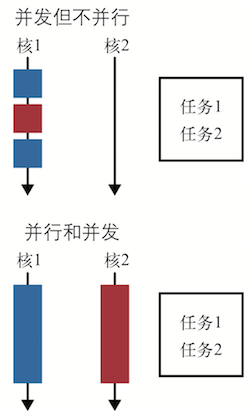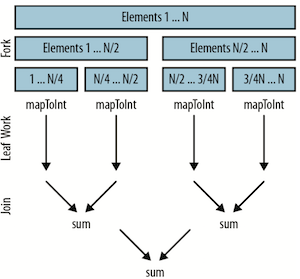# 第 6 章 数据并行化

## 6.1 并行和并发## 6.3 并行化流操作

• 串行化计算专辑曲目长度
``````public int serialArraySum() { return albums.stream()
.flatMap(Album::getTracks)
.mapToInt(Track::getLength)
.sum();
}
``````
• 并行化计算专辑曲目长度
``````    public int parallrlArraySum(List<Album> albums) {
return albums.parallelStream()
.flatMap(Album::getTracks)
.mapToInt(Track::getLength)
.sum();
}
``````

## 6.4 模拟系统

• 使用蒙特卡洛模拟法并行化模拟掷骰子事件
``````    public static final Integer N = 4;

public static Map<Integer, Double> parallelDiceRolls() {
IntFunction<Integer> intFunction = (a) -> (int) (1 + Math.random() * (6 - 1 + 1)) + (int) (1 + Math.random() * (6 - 1 + 1));
double fraction = 1.0 / N;
return IntStream.range(0, N)                   ①
.parallel()                            ②
.mapToObj(intFunction)                 ③
.collect(groupingBy(side -> side,      ④
summingDouble(n -> fraction)));⑤
}
``````

• 通过手动使用线程模拟掷骰子事件
``````//代码太多了，这里就不全部列出来了，具体可参看原文
public class ManualDiceRolls {
private static  nal int N = 100000000;
private  nal double fraction;
private  nal Map<Integer, Double> results; private  nal int numberOfThreads;
private  nal ExecutorService executor; private  nal int workPerThread;
public static void main(String[] args) {
ManualDiceRolls roles = new ManualDiceRolls();
roles.simulateDiceRoles();
}
public ManualDiceRolls() {
fraction = 1.0 / N;
results = new ConcurrentHashMap<>();
}
......
``````

## 6.5 限制

`reduce` 操作的另一个限制是组合操作必须符合结合律。这意味着只要序列的值不变，组合操作的顺序不重要。有点疑惑?别担心!请看如下代码，我们可以改变加法和乘法的顺序， 但结果是一样的。

• 加法和乘法满足结合律
``````(4+2)+1=4+(2+1)=7
(4*2)*1=4*(2*1)=8
``````

## 6.6 性能

• 数据大小 输入数据的大小会影响并行化处理对性能的提升。将问题分解之后并行化处理，再将结果合并会带来额外的开销。因此只有数据足够大、每个数据处理管道花费的时间足够多时，并行化处理才有意义。

• 源数据结构 每个管道的操作都基于一些初始数据源，通常是集合。将不同的数据源分割相对容易， 这里的开销影响了在管道中并行处理数据时到底能带来多少性能上的提升。

• 装箱 处理基本类型比处理装箱类型要快。

• 核的数量 极端情况下，只有一个核，因此完全没必要并行化。显然，拥有的核越多，获得潜在性 能提升的幅度就越大。在实践中，核的数量不单指你的机器上有多少核，更是指运行时 你的机器能使用多少核。这也就是说同时运行的其他进程，或者线程关联性(强制线程 在某些核或 `CPU` 上运行)会影响性能。

• 单元处理开销 比如数据大小，这是一场并行执行花费时间和分解合并操作开销之间的战争。花在流中每个元素身上的时间越长，并行操作带来的性能提升越明显。

• 并行求和
``````private int addIntegers(List<Integer> values) {
return values.parallelStream()
.mapToInt(i -> i)
.sum();
}
``````1. 数据被分成四块。
2. 计算工作在每个线程里并行执行。这包括将每个 `Integer` 对象映射为 `int` 值，然后在每个线程里将 `1/4` 的数字相加。理想情况下，我们希望在这里花的时间越多越好，因为这里是并行操作的最佳场所。
3. 然后合并结果。在上面的并行求和代码中，就是 sum 操作，但这也可能是 `reduce``collect` 或其他终结操作。

• 性能好 `ArrayList`、数组或 `IntStream.range`，这些数据结构支持随机读取，也就是说它们能轻而易举地被任意分解。

• 性能一般 `HashSet``TreeSet`，这些数据结构不易公平地被分解，但是大多数时候分解是可能的。

• 性能差 有些数据结构难于分解，比如，可能要花 O(N) 的时间复杂度来分解问题。其中包括 `LinkedList`，对半分解太难了。还有 `Streams.iterate``BufferedReader.lines`，它们长度未知，因此很难预测该在哪里分解。

## 6.7 并行化数组操作

`Java 8` 还引入了一些针对数组的并行操作，脱离流框架也可以使用 `Lambda` 表达式。像流框架上的操作一样，这些操作也都是针对数据的并行化操作。让我们看看如何使用这些操作解决那些使用流框架难以解决的问题。

• 数组上的并行化操作

parallelPrefix任意给定一个函数，计算数组的和
parallelSetAll使用 Lambda 表达式更新数组元素
parallelSort并行化对数组元素排序

• 使用 for 循环初始化数组
``````public static double[] imperativeInitilize(int size) {
double[] values = new double[size];
for(int i = 0; i < values.length;i++) {
values[i] = i;
}
return values;
}
``````

• 使用并行化数组操作初始化数组
``````    public static double [] parallelInitialize(int size){
double [] values = new double[size];
Arrays.parallelSetAll(values,i ->i);
return values;
}
``````

`parallelPrefix` 操作擅长对时间序列数据做累加，它会更新一个数组，将每一个元素替换为当前元素和其前驱元素的和，这里的“和”是一个宽泛的概念，它不必是加法，可以是任意一个 `BinaryOperator`

• 计算简单滑动平均数
``````    public static double[] simpleMovingAverage(double[] values, int n) {
//不影响原数组，先拷贝一份
double[] sums = Arrays.copyOf(values, values.length); ①
//每一个元素替换为当前元素和其前驱元素的和
Arrays.parallelPrefix(sums, Double::sum);②
int start = n - 1;
//range的作用其实就是产生遍历的下标
return IntStream.range(start, sums.length)③
.mapToDouble(i -> {
//第一个窗口的前一个值不存在因此为0
double prefix = i == start ? 0 : sums[i - n];
return (sums[i] - prefix) / n;④
}).toArray();⑤
}
``````

## 6.8 要点回顾

• 数据并行化是把工作拆分，同时在多核CPU上执行的方式。
• 如果使用流编写代码，可通过调用parallel或者parallelStream方法实现数据并行化 操作。
• 影响性能的五要素是:数据大小、源数据结构、值是否装箱、可用的CPU核数量，以及处理每个元素所花的时间。

## 6.9 练习

1. 下面的代码顺序求流中元素的平方和，将其改为并行处理。
• 顺序处理
``````public static int sequentialSumOfSquares(IntStream range) {
return range.map(x -> x * x).sum();
}
``````
• 并行处理
``````    public static int parallelSumOfSquares(IntStream range) {
return range.parallel()
.map(x -> x * x).sum();
}
``````
1. 下面代码把列表中的数字相乘，然后再将所得结果乘以 5。顺序执行这段程序没有问题，但并行执行时有一个缺陷，使用流并行化执行该段代码，并修复缺陷。
• 把列表中的数字相乘，然后再将所得结果乘以 5，该实现有一个缺陷
``````public static int multiplyThrough(List<Integer> linkedListOfNumbers) {
.reduce(5, (acc, x) -> x * acc);
}
``````
• 修复后的代码
``````    public static int multiplyThrough(List<Integer> linkedListOfNumbers) {
.reduce(1, (acc, x) -> x * acc) * 5;
}
``````
1. 如下代码计算列表中数字的平方和。尝试改进代码性能，但不得牺牲代码质量。 只需要一些简单的改动即可。
• 求列表元素的平方和，该实现方式性能不高
``````public int slowSumOfSquares() {
``````public int slowSumOfSquares() {